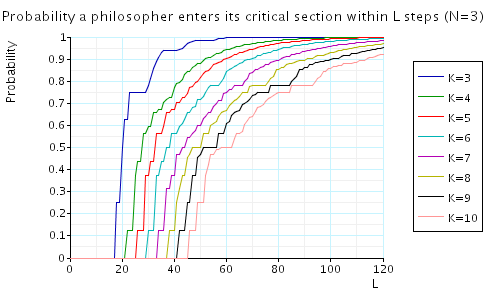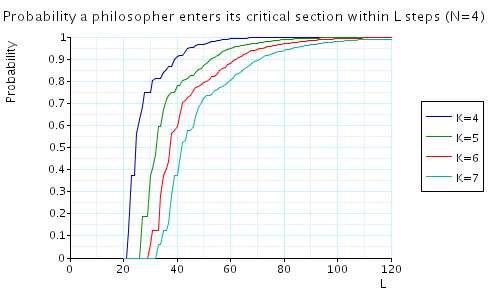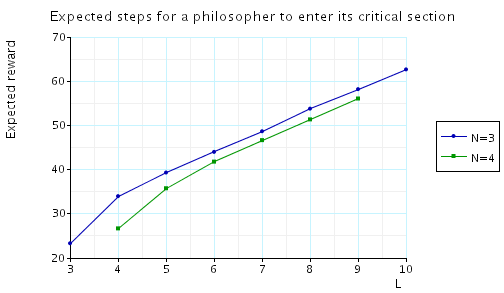# Randomised Dining Philosophers

(Lynch, Saias and Segala)

### Introduction

This case study is based on Lehmann and Rabin's solution to the well known dining philosophers problem [LR81] and the analysis of this algorithm by Lynch, Saias and Segala [LSS94]. In particular, Lynch, Saias and Segala consider proving time bounds for this algorithm by making the following assumption:

• "A philosopher in its trying region cannot wait more than one time unit before it is given a chance to perform a step."

Under this assumption, Lynch, Saias and Segala proved that the following property holds:

• "If some philosopher is in its trying region, then with probability at least 1/8, some philosopher enters its critical section within 13 time units."

However, since we only model discrete time we cannot make the assumption given above. Instead we make the following assumption:

• "If a philosopher is in its trying region then at most K transitions can occur between it being scheduled."
• This is a stronger assumption than that of Lynch, Saias and Segala, and hence we consider a smaller class of adversaries or schedulers. As a result, we can prove stronger properties than that given above (dependent on the value of K).

The model is represented as an MDP. In the following, N denotes the number of philosophers and K is as above, i.e. the maximum number of transitions that can occur between a philosopher in its trying region being scheduled. By way of example, the PRISM source code for the case where N=3 is given below.

```// Lehmann-Rabin algorithm [LR82] (dining philosophers)
// we suppose an action takes 1 time unit
// an a process can wait at most K time units before making a transition
// gxn/dxp 01/02/00

mdp

// CONSTANTS
const K;

// COUNTER FORMULAE
// ci number of steps since process last moved
// removing trying and remainder states since
// these are controlled by the process not the adversary

// process can go if it does not stop one of the other processes round counter reaching K+1
// added complication if two processes counter equals K-1, then if neither makes a transition
// both reach K, and hence one must reach K+1 which we must disallow
formula can1 = !((c2=K) | (c3=K) | ((c2=K-1) & (c3=K-1)));
formula can2 = !((c1=K) | (c3=K) | ((c1=K-1) & (c3=K-1)));
formula can3 = !((c1=K) | (c2=K) | ((c1=K-1) & (c2=K-1)));

// when a process moves the counters of the other processes increase
// but only when they are not in trying or remainder
formula count1  = (p1!=13) & (p1!=0);
formula count2  = (p2!=13) & (p2!=0);
formula count3  = (p3!=13) & (p3!=0);
formula ncount1 = (p1=13) | (p1=0);
formula ncount2 = (p2=13) | (p2=0);
formula ncount3 = (p3=13) | (p3=0);

module counter

c1 : [0..K];
c2 : [0..K];
c3 : [0..K];

// process 1 moves
[s1] count2  & count3  & can1 -> (c1'=0) & (c2'=c2+1) & (c3'=c3+1);
[s1] ncount2 & count3  & can1 -> (c1'=0) & (c3'=c3+1);
[s1] count2  & ncount3 & can1 -> (c1'=0) & (c2'=c2+1);
[s1] ncount2 & ncount3 & can1 -> (c1'=0);
// process 2 moves
[s2] count1  & count3  & can2 -> (c2'=0) & (c1'=c1+1) & (c3'=c3+1);
[s2] ncount1 & count3  & can2 -> (c2'=0) & (c3'=c3+1);
[s2] count1  & ncount3 & can2 -> (c2'=0) & (c1'=c1+1);
[s2] ncount1 & ncount3 & can2 -> (c2'=0);
// process 3 moves
[s3] count1  & count2  & can3 -> (c3'=0) & (c1'=c1+1) & (c2'=c2+1);
[s3] ncount1 & count2  & can3 -> (c3'=0) & (c2'=c2+1);
[s3] count1  & ncount2 & can3 -> (c3'=0) & (c1'=c1+1);
[s3] ncount1 & ncount2 & can3 -> (c3'=0);

endmodule

// PHILOSOPHER 1
// atomic formule
// left fork and right fork free resp.
formula lfree = p2>=0&p2<=4|p2=6|p2=11|p2=13;
formula rfree = p3>=0&p3<=3|p3=5|p3=7|p3=12|p3=13;

module phil1

p1 : [0..13];

[s1] (p1=0) -> (p1'=0); // try
[s1] (p1=0) -> (p1'=1);
[s1] (p1=1) -> 0.5 : (p1'=2) + 0.5 : (p1'=3); // flip
[s1] (p1=2) &  lfree -> (p1'=4); // wl and l free
[s1] (p1=2) & !lfree -> (p1'=2); // wl and l taken
[s1] (p1=3) &  rfree -> (p1'=5); // wr and r free
[s1] (p1=3) & !rfree -> (p1'=3); // wr and r taken
[s1] (p1=4) &  rfree -> (p1'=8); // l and r free
[s1] (p1=4) & !rfree -> (p1'=6); // l and r taken
[s1] (p1=5) &  lfree -> (p1'=8); // r and l free
[s1] (p1=5) & !lfree -> (p1'=7); // r and l taken
[s1] (p1=6)  -> (p1'=1); // nr
[s1] (p1=7)  -> (p1'=1); // nl
[s1] (p1=8)  -> (p1'=9); // pre_crit
[s1] (p1=9)  -> (p1'=10); // crit
[s1] (p1=10) -> (p1'=11); // exit
[s1] (p1=10) -> (p1'=12);
[s1] (p1=11) -> (p1'=13); // put down left first
[s1] (p1=12) -> (p1'=13); // put down right first
[s1] (p1=13) -> (p1'=0); // remainder
[s1] (p1=13) -> (p1'=13);

endmodule

// construct further processes through renaming
module phil2=phil1 [p1=p2, p2=p3, p3=p1, s1=s2] endmodule
module phil3=phil1 [p1=p3, p2=p1, p3=p2, s1=s3] endmodule

// reward structure - number of steps
rewards "steps"
[s1] true : 1;
[s2] true : 1;
[s3] true : 1;
endrewards
```

### Model Statistics

The table below shows statistics for the MDPs we have built for different values of the constants N and K. The tables include:

• the number of states and transitions in the MDP representing the model;
• both the number of nodes and leaves of the MTBDD which represents the model;
• the construction time which equals the time taken for the system description to be parsed and converted to the MTBDD representing it, and the time to perform reachability, identify the non-reachable states and filter the MTBDD accordingly;
• the number of iterations required to find the reachable states (which is performed via a BDD based fixpoint algorithm).

 N: K: Model: MTBDD: Construction: States: Transitions: Nodes: Leaves: Time (s): Iter.s: 3 4 28,940 77,292 11,805 3 1.465 27 5 47,204 135,636 15,235 3 1.630 27 6 69,986 211,344 18,691 3 2.358 28 7 97,298 304,152 21,063 3 2.757 29 8 129,254 414,318 25,014 3 3.649 30 9 165,746 541,908 27,072 3 3.534 31 10 206,864 686,820 28,506 3 5.024 32 4 4 729,080 1,876,960 76,041 3 17.87 34 5 1,692,144 5,035,016 121,758 3 32.17 42 6 3,269,200 10,704,752 179,714 3 50.34 36 7 5,609,336 19,662,904 242,666 3 75.78 37 8 8,865,024 32,653,704 314,314 3 57.07 38 9 13,192,376 50,438,968 374,738 3 98.06 39 10 18,740,512 73,785,912 425,481 3 102.5 40

### Model Checking

When model checking, we have the following requirements:

• Scheduler-Liveness: for each time t and any philosopher Pi, there exists a time t' > t in which Pi makes a move.
• Bounded-Waiting: if a philosopher is in its trying region, then at most m transitions can occur between it being scheduled.

These correspond to the conditions on the adversary/scheduler with the first being imposed by model checking against only fair adversaries.

The properties we have model checked are given below.

```const int L; // discrete time bound

label "trying" =((p1>0) & (p1<8))  | ((p2>0) & (p2<8)) | ((p3>0) & (p3<8)); // philosopher in its trying region
label "entered" =((p1>7) & (p1<13)) | ((p2>7) & (p2<13)) | ((p3>7) & (p3<13)); // philosopher in its critical section

// lockout free
"trying" =>  P>=1 [ true U "entered" ]

// bounded until: minimum probability (from a state where a process is in its trying region) that some process enters its critical section within k steps
Pmin=? [ true U<=L "entered" {"trying"}{min} ]

// expected time: maximum expected time (from a state where a process is in its trying region) that some process enters its critical section
Rmax=? [ F "entered" {"trying"}{max} ]
```